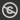Science, Maths & Technology
Free course

# Introduction to analysis## Course reviews

This free course is an introduction to analysis which looks at real numbers and their properties, with a particular emphasis on inequalities. Section 1 starts by revising rational numbers and their decimal representations. Then, real numbers are introduced as infinite decimals. Section 2 looks at rules for manipulating inequalities and finding the solution set of an inequality. Section 3 looks at various techniques for proving inequalities. Section 4 introduces the concept of a least upper bound. Section 5 looks at how least upper bounds can be used to define arithmetic operations on the set of real numbers.

## Course learning outcomes

After studying this course, you should be able to:

• find a rational and an irrational number between any two distinct real numbers
• solve inequalities by rearranging into simpler equivalent forms and solve inequalities involving modulus signs
• state and use the Triangle Inequality and use the Binomial Theorem and mathematical induction to prove inequalities which involve an integer n
• use the strategies introduced for determining least upper bounds and greatest lower bounds
• explain how the least upper bound property is used to define arithmetical operations with real numbers and explain the meaning of rational powers.

First Published: 08/12/2017

Updated: 17/04/2018

Skip Rate and Review